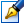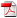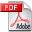##Cubic differential systems with invariant lines of total multiplicity eight

 Author: Bujac Cristina Degree: doctor of Speciality: 01.01.02 - Differential equations Year: 2016 Scientific adviser: Nicolae Vulpedoctor habilitat, professor, Institute of Mathematics and Computer Science of the ASM Institution: Institute of Mathematics and Computer Science of the ASM

### Status

The thesis was presented on the 31 March, 2016
Approved by NCAA on the 3 June, 2016

### Abstract0.53 Mb / in romanian0.53 Mb / in english

### Thesis

CZU 517.9251.57 Mb / in english
165 pages

### Keywords

cubic differential system, affine invariant polynomial, invariant straight line, multiplicity of a line, configuration of invariant straight lines, perturbed system

### Summary

The language of the Thesis is English. It comprises 154 base pages and has the following structure: Introduction, 4 Chapters, General Conclusions and Recommendations, Bibliography with 140 References. Research outcomes were reflected in 19 scientific publications. Keywords: cubic differential system, affine invariant polynomial, invariant straight line, multiplicity of a line, configuration of invariant straight lines, perturbed system.

Field of study: Qualitative Theory of Dynamical Systems, Invariant Theory of Differential Equations.

The purpose and objectives: to give a full classification for the family of cubic systems with invariant straight lines of total multiplicity eight; this classifications supposes the de- tection of all possible configurations of invariant lines for this family and the construction of affine invariant criteria for the realization of each one of the detected configurations.

Novelty and scientific originality. In our Thesis for the first time there are constructed all the possible configurations of invariant lines of total multiplicity eight for cubic systems. Our set of configurations contains as particular cases all the configurations detected by other authors in special cases. Additionally we give necessary and sufficient conditions for the realization of each one of the corresponding configurations. Moreover we completed the classification of Llibre and Vulpe detecting a new class of cubic systems with invariant lines of total multiplicity nine.

The main scientific problem which is solved in this Thesis consistsin classifying the whole family of cubic differential systems possessing invariant lines of total multiplicity eight according to configurations of these lines; this classification is very helpful for obtaining the complete topological classification of this family and is useful for the study of integrability of these systems.

The significance of theoretical and practical values of the work. The obtained in this thesis results concerning cubic systems with invariant lines of total multiplicity eight represent an important step in algebraic and geometric studies of cubic differential systems.

Implementation of the scientific results. They could be applied: (i) as a basis for determining of the first integrals of such systems; (ii) for further investigations of cubic systems with invariant lines of total multiplicity less then 8; (iii) in the study of some mathematical models which describe processes in physics, chemistry, medicine and so on; (iv) as a support for teaching courses in higher education.

### Summary

1. ADVANCES IN THE STUDY OF CUBIC SYSTEMS WITH INVARIANT STRAIGHT LINES
• 1.1. Polynomial differential systems with invariant straight lines
• 1.2. Invariant algebraic curves in the study of integrability of planar polynomial systems
• 1.3. Invariant theory of polynomial differential systems in the problem of classification
• 1.4. Conclusions on Chapter 1

2. CUBIC SYSTEMS WITH INVARIANT LINES OF TOTAL MULTIPLICITY EIGHT AND FOUR DISTINCT INFINITE SINGULARITIES
• 2.1. Preliminaries
• 2.1.1. Main invariant polynomials associated to configurations of invariant lines
• 2.1.2. The scheme of the proofs of the Main Theorems
• 2.2. Classification of cubic systems according to their configurations of invariant lines
• 2.2.1. Cubic systems with four distinct real infinite singularities
• 2.2.2. Cubic systems with 2 real and 2 complex infinite singularities
• 2.3. Invariant criteria for the realization of the configurations with four distinct infinite singularities
• 2.4. Conclusions on Chapter 2

3. CUBIC SYSTEMS WITH INVARIANT LINES OF TOTAL MULTIPLICITY EIGHT AND EITHER THREE DISTINCT OR ONE INFINITE SINGULARITIES
• 3.1. Cubic systems with three distinct infinite singularities
• 3.1.1. Construction of normal forms and of the corresponding configurations of invariant lines
• 3.1.2. Invariant criteria for the realization of the configurations with three distinct infinite singularities
• 3.1.3. Perturbations of normal forms
• 3.2. Cubic systems with exactly one infinite singularity
• 3.2.1. Construction of cubic homogeneities
• 3.2.2. Construction of canonical forms and of the corresponding configurations of invariant lines
• 3.2.3. Invariant criteria for the realization of the configurations with exactly one infinite singularity
• 3.2.4. Perturbations of canonical forms
• 3.3. Conclusions on Chapter 3

4. CUBIC SYSTEMS WITH INVARIANT LINES OF TOTAL MULTIPLICITY EIGHT AND TWO DISTINCT INFINITE SINGULARITIES
• 4.1. Construction of canonical forms and of the corresponding configurations of invariant lines
• 4.2. Invariant criteria for the realization of the configurations with two distinct infinite singularities
• 4.3. Perturbations of canonical forms
• 4.4. One new class of cubic systems with maximum number of invariant lines
• 4.5. Conclusions on Chapter 4

GENERAL CONCLUSIONS AND RECOMMENDATIONS